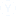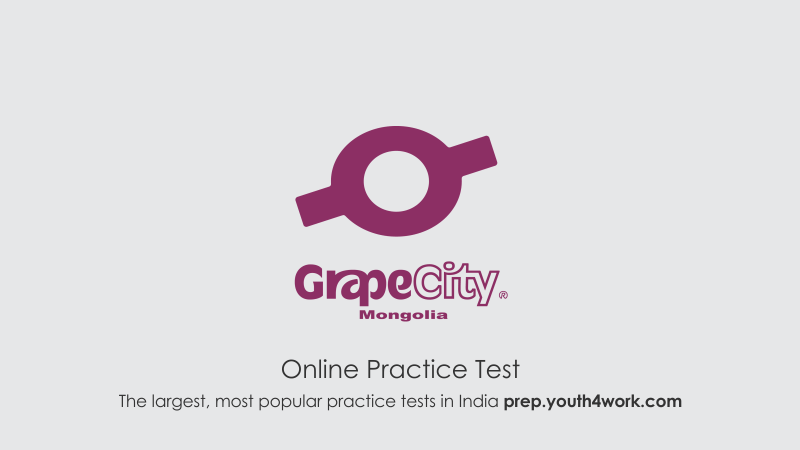which topics are important for the written exam?
need syllabus
• SC
• Suneeta
• 28 ago.
• 1493 Visto
• 2 Respuestas2 Respuestas
1-20 of  5000
2 Respuestas
•
Syllabus
Written Exam- This section consists questions from basic mathematics like Algebra, Permutation & combination, Algebra, Arithmetic, Probability, Speed & Distance, Algebra etc. and Programming.
Technical Questions- This section consists topics from C, C++, Java, OS etc.

• Hi suneeta, how are you?
all topics are important if you are preparing for such kind of exam. But, sharing some important points with you.
·         Aptitude(Quantitative +Verbal +Technical): This section consists of questions based on data interpretation, graphs, blood relations, statement reasoning, etc. Also, there are questions from basic mathematics like time, speed & distance, algebra, arithmetic, probability, geometry, permutation & combination, etc. Further, there are questions from English language like grammar usage, fill in the blanks, etc. Technical questions are generally from topics like C, C++, Java, OS, etc.
·         Programming: In this section consists 2 questions. One question is of your choice and other question is based on printing some pattern.Otras discusiones relacionadasPractica simulacro de prueba para
Grapecity